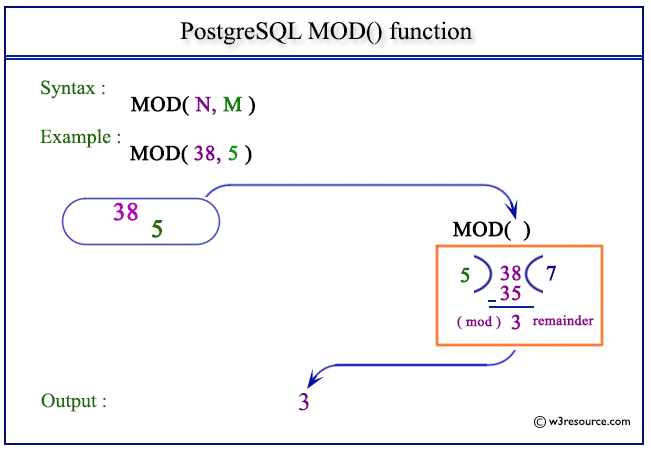# PostgreSQL MOD() function

## MOD() function

The PostgreSQL mod() function is used to return the remainder of a division of two numbers, as specified in the argument.

Syntax:

```mod()
```

PostgreSQL Version: 9.3

Pictorial presentation of PostgreSQL MOD() functionExample: PostgreSQL MOD() function

Code:

``````SELECT MOD(38,5) AS "Remainder";
```
```

Sample Output:

``` Remainder
-----------
3
(1 row)

```

Example: PostgreSQL MOD() function using negative value

Code:

``````SELECT MOD(-38,5) AS "Remainder";
```
```

Sample Output:

``` Remainder
-----------
-3
(1 row)

```

Previous: LOG function
Next: PI function

﻿